Methods of Pricing Material Issues - Material Cost, Cost Accounting

# Methods of Pricing Material Issues - Material Cost, Cost Accounting - Notes | Study Cost Accounting - B Com

 1 Crore+ students have signed up on EduRev. Have you?

Method of Pricing of Material Issues
When materials are issued to production department, a difficulty arises regarding the price at which materials issued are to be charged. The same type of material may have been purchased in different lots at different times at several different prices. This means that actual cost can take on several different values and same method of pricing the issue of materials must be selected.
There are numerous methods of pricing issues. They may be classified as follows:

I. Cost Price Methods

1. Specified Price
2. First-in First-out (FIFO)
3. Last-in First-out (LIFO)
4. Highest-in First-out (HIFO)
5. Base Stock

II. Average Price Method

1. Simple Average
2. Weighted Average
3. Periodic Simple Average
4. Moving Simple Average
5. Moving Weighted Average

III. Notional Price Methods

1. Standard Price
• Current Standard
• Basic Standard
2. Inflated Price
3. Market Price
• Replacement Price
• Realisable Price

I. Cost Price Methods
(a) Specified Price (Identifiable) Method
Sometimes materials are purchased to be utilised in a particular job or issues can be identified with a particular receipt. In these cases, the actual purchase price can be charged. This method can be adopted when prices are stable or when the materials are covered by price control orders. This method has limited application only.

(b) First-in First-out (FIFO) Method
This method is based on the assumption that materials which are purchased first are issued first. It uses the price of the first batch of materials purchased for all issues until all units from this batch have been issued. In other words the materials are issued at the oldest cost price listed in the stores ledger account and thus, the materials in stock are valued at the price of the latest purchases. It should be noted that the assumption of FIFO is only for accounting purpose i.e. the physical flow of materials need not necessarily be in the order of the flow of cost, though normally materials would be expected to move out of stock on approximately a FIFO basis because oldest stocks are usually used up first.

Example
Receipts
20th Oct. 500 kgs. @ 5.00 per kg.
23rd Oct. 250 kgs. @ 5.50 per kg.

Issues
25th Oct. Issue of 600 kgs. will be valued as follows:
500 kgs. @ Rs. 5 per kg.
100 kgs. @ Rs. 5.50 per kg.

(i) It is a good inventory management system since the oldest units are used first and inventory consists of the latest stock.
(ii) It is logical.
(iii) It is easy to understand and operate.
(iv) It facilitates inter-firm and intra-firm comparisons.
(v) Valuation of inventory and cost of finished goods is consistent and realistic.

(i) The cost of production is not linked to the current prices.
(ii) If prices are rising, production cost is understated. But if stock turnover rate is high, the inventory will reflect current prices. The effect of current market prices is not revealed in issues when prices are rising.
(iii) It does not present the true picture when many lots are purchased at different prices.
The calculation become complicated.
(iv) The pricing of material returns is difficult.
(v) High inflation creates problems in replacing used materials, this aspect is not dealt with in FIFO.
(vi) Usually more than one price has to be adopted for a particular issue.
(vii) Cost comparisons between two batches of production becomes difficult when issues are priced differently.

(c) Last-in First out (LIFO) Method
The principle adopted is that the materials used in production is from the latest purchase. The inventory is priced at the oldest costs. As the method applies the current cost of materials to the cost of units, it is also known as the replacement cost method. It is the most significant method in matching cost with revenue in the income determination procedure.

Example
Assuming the same facts as given under FIFO, the issues will be valued as follows:
250 kgs. @ Rs.5.50 per kg.
350 kgs. @ Rs. 5.00 per kg.

(i) It is simple and useful when transaction are few.
(ii) It is a good method of avoiding tax.
(iii) It is a systematic method. It matches current costs with current revenues in a better way.
(iv) It reveals real income in times of rising prices.
(v) It minimises unrealised inventory gains and losses and tends to stabilise reported operation profits especially when the industry is prone to sharp price fluctuations.

(i) When rates of material receipts are highly fluctuating, the method becomes complicated.
(ii) More than one price may have to be adopted for an issue.
(iii) Cost of different batches vary greatly, making inter-firm and intra-firm comparison difficult.
(iv) The stocks require to be adjusted during falling prices.
(v) Unless purchases and sales occur in equal quantities the current costs cannot be easily matched with current revenue.
(vi) The company can time the purchases to cause high or low costs thus changing reported income at will.
(vii) Existing profit sharing and bonus can be effected by an accounting change. Employees will have difficulty in understanding the cause for these changes.

(d) Highest-in First-out (HIFO) Method
The principle adopted is that costliest materials are issued first, Inventory is valued at the lowest possible price. The method requires detailed records. It is mainly used for monopoly products or cost plus contracts. When stocks are undervalued, a secret reserve is created.

(e) Base Stock Method
A certain minimum stock of a material is always carried and is priced at the original cost (usually at the lowest purchase price). The portion of stock above this level is issued and priced under any one of the methods. The disadvantages of this method is that the stock may be under valued and hence the computation of return on capital will not be reliable.

II. Average Price Methods
(a) Simple Average Method
The simple average is the average of prices ignoring the quantities involved. It can be used when the prices are normally stable and the stocks purchased are in equal quantities or the stock value is small. It is calculated by dividing the total rates of materials by the number of rates of prices. A new average is worked out after every receipt.

Example:
Assuming the facts given in FIFO the average will be: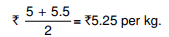(b) Weighted Average Method
In this method, the total quantities and total costs are taken into account while calculating the average price. It is calculated after every purchase by adding the quantity received to the stock in hand and the cost of this purchase to the cost of stock in hand. The total cost is divided by the total quantity to arrive at the value. This method avoids price fluctuations and reduces the number of calculations and gives an acceptable figure for stock.

Example
The weighted average will be calculated as follows (with previously given data):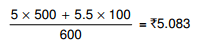(i) It is logical and consistent.
(ii) Changes in prices do not affect issues and inventory.
(iii) The values reflect actual costs.

(i) It involves considerable amount of clerical work.
(ii) When prices change frequently, it is inconvenient and complex.
(iii) As it is not the actual price, it is not realistic.

(c) Periodical Simple Average Method
Some companies may price materials by taking average of the prices of all receipts during a period, e.g., a month, a week, etc. for the subsequent period. Only those prices - relevant to the period is taken into account. Purchases made during the period and closing stock are taken into account.

Example
The receipts during the month were at the rates of ` 5, ` 5.50, ` 6 and ` 4.50. The periodic simple average will be: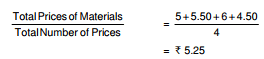(i) Pricing of issues ignores heavy fluctuations in price during the current period.
(ii) it is not an exact cost method.
(iii) It involves heavy clerical work.

(d) Periodic Weighted Average Method
The average price is calculated periodically and not every time the material is received. It is calculated by dividing the total value of materials purchased during a period by the total quantity purchased.

Example
If the total receipts during a month is 1,000 kg. costing `25,000, the periodic weighted average will be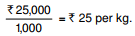(i) Clerical costs are reduced.
(ii) It is useful in process costing.
(iii) The issue price is not affected by short-term fluctuations.

(i) At the end of the accounting period, heavy clerical work is involved.
(ii) Violent fluctuations are ignored till the end of the period.
(iii) Closing stock can be erroneously valued and nil stock may have a residual value.

(e) Moving Simple Average Method
In this method, periodic simple average prices are further averaged. By dividing periodic average prices by the number of periods taken, the moving average is calculated. The period chosen should cover the period in which the material is issued. The value of closing stock may be under valued or over valued. When prices are rising, the issue price worked out is lower than the periodic average prices for the period concerned and vice versa.

Example

 Month Periodic Average Price (Rs) Moving Average Price  (Rs) January 2.50 February 2.60 2.60 March 2.70 2.72 April 2.85 2.85 May 3.00 3.03 June 3.25

(f) Moving Weighted Average Method
The material issue price is calculated by dividing the total of the periodic weighted average prices for a number of periods by the total number of such periods.

III. Notional Price Methods
(a) Standard Price Method
The price of issues for each item is pre-determined for a stated period taking into account all the factors affecting price, e.g., market trends, transportation costs, etc. Standard prices are determined for each material. All issues and inventory are kept at the standard price. These should be revised from period to period.

Standard can be basic or current standard. The basic standard is fixed for long periods and it gives the ideal price. It assists forward planning. Current standard keeps costs of the products adjusted to prevailing trends in markets. Basic standard on the other hand, helps to study trends in production costs over a period.

The difference between standard and actual is transferred to the purchase price variance account.

(i) It simplifies accounting as only quantities are recorded.
(ii) As only one rate is adopted, inconsistency is avoided.
(iii) It helps to determine purchase efficiency. If actual cost is more than the standard than there is unfavourable purchasing efficiency and vice versa.
(iv) It is simple to operate.
(v) It provides stability to the costing system.

It does not reflect the actual or expected cost but only a target.

(b) Inflated Price Method
Inflated price includes carrying costs, losses due to evaporation etc. It aims to recover full costs of materials purchased.

(c) Market Price Method
Materials may be issued at the replacement price. The replacement price is the cost of the same type of materials in the market at any given time.

(i) It measures results correctly and accurately as current revenues are matched against current costs.
(ii) It differentiates between holding gains and operating gains.
(iii) A realistic and competitive selling price can be determined.

(i) In the absence of a market price, replacement price cannot be determined.
(ii) As it is not based on actual cost, they may increase the confusion and complication in accounting.
The replacement price is used in respect of items used in manufacturing whereas the realisable price is used for items kept in stock.
The realisable price is useful for calculating the issue price of obsolete and slow-moving stores. If issues are priced at current market price, price reduced due to bulk purchases, are not reflected.
The market price method introduces elements of uncertainty and involves excessive classical labour to maintain records of latest prices for various items.

Selection of Material Pricing Method
The various method of pricing issues have merits and demerits. The choice of any method depends on many factors which can be summarised as under :

1. The frequency of purchases.
2. Price fluctuations and its range.
3. Method of stock valuation.
4. Customs and practices followed in the industry, whether uniform costing system is being followed.
5. Stock turnover rate.
6. Percentage cost of raw materials to total cost of products.
7. Economic order quantity.
8. Effect of pricing method on tax payable.
9. The accuracy required and the accuracy which would be obtained.
10. Clerical work involved.
12. Traceability of issue to purchase lot.
13. Frequency of receipts and issues.
14. Whether standard costing system is adopted.
16. The possibility of using different methods for different classes of items.

In addition to the above, the following factors have to be satisfied:
(i) The purchase cost is covered.
(ii) The issue price reflects the market price,
(iii) There is no significant variation in cost from period to period, and
(iv) The system does not necessitate heavy adjustment at the time of valuing closing stock.

Illustration 8
Following is the information by XYZ company Ltd. Related to first week of December, 2013:
The transactions in connection with the materials are as follows:

 Days Receipts Issues Units Rate per unit Rs (units) 1st 40 15.00 2nd 20 16.50 3rd - - 30 4th 50 17.10 - 5th - - 20 6th - - 40

Calculate the cost of materials issued under (i) FIFO METHOD; (ii) LIFO method; and (iii) Weighted average method of issue of materials nad value of closing stock under the above methods.
Solution:
(i) Cost of materials issued and value of closing stock under FIFO Method

 Rs. Rs. December 3, 2013: issued 30 units @ Rs.15 per unit 450 December 5, 2013: issued 10 units @ Rs. 15 150 issued 10 units @ Rs 16.50 165 315 December 6, 2013: issued 10 units @Rs. 16.50 165 Issued 30 units @ Rs 17.10 513 678 Closing stock: 20 units @ Rs. 17.10 342

(ii) Cost of materials issued and value of closing stock under LIFO Method:

 Rs. Rs. December 3, 2013: issued 20 units @ Rs. 16.50 330 Issued 10 units @ Rs15.00 150 480 December 5, 2013: issued 20 units @ Rs.17.10 342 December 6, 2013: issued 30 units @ Rs. 17.10 513 Issued 10 units @ Rs 15.00 150 663 Closing stock: 20 units @ Rs 15.00 300

(iii) Cost of materials issued and value of closing stock under Weighted Average Method

 Rs. December 3, 2013: issued 30 units =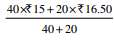i.e. 30 x Rs15.50 465 December 5, 2013: issued 20 units @ Rs. 16.50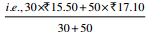330 December 6, 2013: issued 40 units @ Rs. 16.50 660 Closing stock: 20 units @ Rs. 16.50 330

Material Problems with Solutions
1. Cost Sheet Problems with Solutions (5 Problems):
Cost Sheet Problem 1:

(a) A manufacturer uses 200 units of a component every month and he buys them entirely from outside supplier. The order placing and receiving cost is Rs.100 and annual carrying cost is Rs.12. From this set of data calculate the Economic Order Quantity.
(b) P. Ltd. uses three types of materials A, B & C for production of X, the final product.
The relevant monthly data for the components are as given below: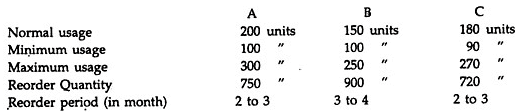Calculate for each component
(a) Re-order level
(b) Minimum level
(c) Maximum level
(d) Average stock level
Solution.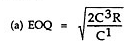where C3 = ordering cost, C1 = Carrying cost , R = Annual requirements. i.e.
= 200 x 12 = 2400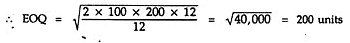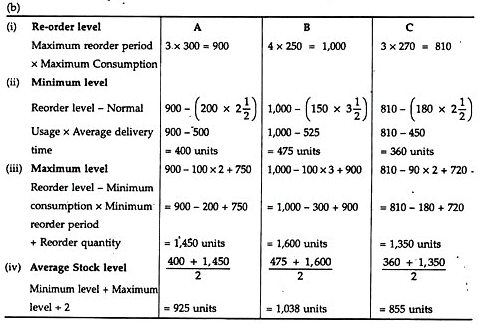Cost Sheet Problem 2:
(a) From the following data for the last 7 days, compute the Average Stock Level for a component: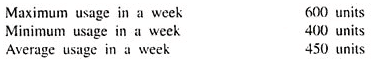Time-lag procurement of materials: Maximum 4 days. Minimum 2 days. Re-order quantity = 1,500 units.
(b) You also ascertain the Danger Stock Level from the above information.
Solution:
(a) Average Stock Level = Minimum Level* + ½ (Re-order quantity)
= 1.050 units + ½ × 1,500 units = 1,800 units
(b) Danger Stock Level = Average usage x Minimum Re-order Period
= 450 units × 2 = 900 units
Working Note:
(i) Re-order Level = Maximum usage x Maximum Re-order period
= 600 × 4 = 2,400 units
(ii) Minimum Level = Re-order Level – (Average usage in a week x Average delivery period)
= 2,400 – (450 × 4 + 2/2)
= 2,400 – 1,350 = 1,050 units
Problem 2(a):
Compute the re-order level, minimum level, maximum level, average stock level for components A and B based on the following data: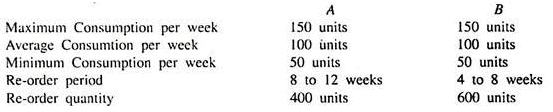Solution: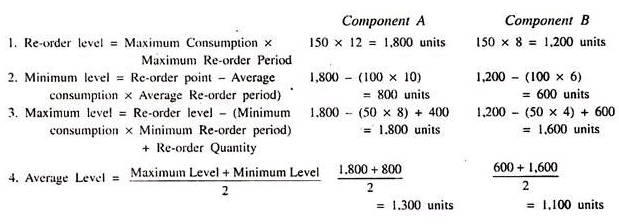Problem 2(b):
ZEE is a product manufactured out of three raw materials M, N and Q. Each unit of ZEE requires 10 kg, 8 kg and 6 kg of M, N and Q, respectively. The re-order levels of M and N are 15,000 kg and 10,000 kg, respectively, while the minimum level of Q is 2,500 kgs. The weekly production of ZEE varies from 300 to 500 units, while the weekly average production is 400 units.
You are required to compute:
(i) The minimum stock level of M.
(ii) The maximum stock level of N; and
(iii) The re-order level of Q.
The following additional data are given: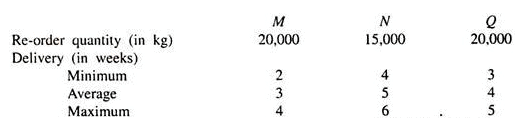Solution:
(i) Minimum stock level of M:
Minimum level = Re-order level – (Normal Consumption x Normal Delivery period)
= 15,000 kg – (400 units x 10 kg × 3 weeks) = 15,000 – 12,000 = 3,000 kg.
(ii) Maximum Stock Levels of N:
Maximum level = Re-order level + Re-order Quantity
– (Minimum Consumption x Normal Minimum period)
= 10,000 kg + 15,000 kg. – (300 x 8 x 4) kg
= 25,000 – 9,600 = 15,400 kg.
(iii) Re-order Level of Q:
Re-order level = (Maximum consumption × Maximum period)
= (500 × 5 × 6) = 15,000 kg.

Problem 2(c):
In a factory component A is used as follows:
Normal usage – 50 kg per week
Minimum usage – 25 kg per week
Maximum usage – 75 kg per week
Re-order quantity 300 kg.
Re-order period 4 to 6 weeks.
Calculate for component A:
(i) Re-order level,
(ii) Maximum level.
(iii) Minimum level; and
(iv) Average stock level.
Solution:
(i) Re-order level = Maximum usage × Maximum period
= 75 × 6 = 450 kg
(ii) Maximum level = Re-order level + Re-order quantity – (Minimum usage × Minimum time)
= 450 + 300 – (25 × 4) = 650 kg.
(iii) Minimum level = Reorder level – (Normal usage × Average time)
= 450 – (50 × 5) = 200 kg.
(iv) Average stock level = Maximum level + Minimum level/2
= (650 + 200/2)kg = 425 kg.

Cost Sheet Problem 3:
Find the Economic Order Quantity from the following data: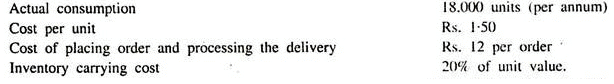Solution:
EOQ = √2AB/CS
= √2 × 18,000 × 12 × 100/1.50 × 20
√4,32,00,000/30
= √14,40,000 = 1,200 units
where, EOQ – Economic Order Quantity
A = Annual Consumption
B = Ordering Cost
C = Cost per unit
S = Inventory Carrying Cost.

Cost Sheet Problem 4:
Find out the Economic Order Quantity and Order Schedule for raw materials and packing materials with the following data given to you:
1. Cost of ordering: Raw Materials = Rs. 1,000 per order
Packing Materials = Rs. 5,000 per order
2. Cost of holding inventory: Raw Materials = 1 paisa per unit p.m.
Packing Materials = 5 paise per unit p.m.
3. Production rate: 2,00,000 units per month
Solution:
(i) In the case of Raw Materials:
EOQ = √2AB/CS
= √2 × 2,00,000 × 1,000 × 100/1
= 2,00,000 units
One order per month = 2,00,000 units
where, EOQ = Economic Order Quantity
A = Annual Consumption
B = Ordering or buying Cost per order
C = Cost per unit
S = Carrying Cost or Storage Cost
(ii) In the case of Packing Materials:
EOQ = √2AB/CS = √2 × 2,00,000 × 5,000 × 100/5
= 2,00,000 units
One order per month = 2,00,000 units.

Cost Sheet Problem 5:
Company uses 5,120 units of a component in a year. The purchase price per unit of the component is Rs. 5.00 and the carrying cost including interest is estimated at 20% of the average inventory investment on annual basis. The cost of placing an order and processing the delivery is Rs. 10.
(a) Ascertain the economic order quantity and the number of orders each of the economic order quantity, to be placed for the component in a year.
(b) Assuming that the average daily consumption of the above component is 14 units and that the normal lead time is 15 days, calculate the ordering level for the component, where safety stock is considered to be equal to 25 days’ consumption. State why ordering level is fixed.
Solution:
EOQ = √2AB/CS = √2 × 5,120 × 10 × 100/5 × 20 = 320 units
where, EOQ = Economic Order Quantity
A = Annual usage = 5,120 units
B = Cost of placing an order and processing delivery = Rs. 10
C = Cost per unit = Rs. 5
S = Carrying cost including interest = 20%
No. of orders = Annual usage/Economic Order Quantity
= 5,120 units/320 units = 16 times
Cost per order of EOQ = 320 units × Rs. 5 + Rs. 10 + 20% of (320 units × Rs. 5 + Rs. 10)
= Rs. 1,932
(b) Ordering Level = Minimum Stock Level + Average consumption day × Normal lead time
= (14 × 25) + (14 × 15)
= 350 + 210 = 560 units.

The document Methods of Pricing Material Issues - Material Cost, Cost Accounting - Notes | Study Cost Accounting - B Com is a part of the B Com Course Cost Accounting.
All you need of B Com at this link: B Com

## Cost Accounting

107 videos|139 docs
 Use Code STAYHOME200 and get INR 200 additional OFF

## Cost Accounting

107 videos|139 docs

Track your progress, build streaks, highlight & save important lessons and more!

,

,

,

,

,

,

,

,

,

,

,

,

,

,

,

,

,

,

,

,

,

,

,

,

;